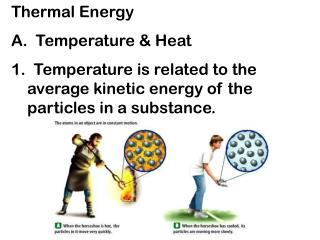DownloadDownload PresentationThermal Energy A. Temperature & Heat

# Thermal Energy A. Temperature & Heat

Télécharger la présentation## Thermal Energy A. Temperature & Heat

- - - - - - - - - - - - - - - - - - - - - - - - - - - E N D - - - - - - - - - - - - - - - - - - - - - - - - - - -
##### Presentation Transcript

1. Thermal Energy A. Temperature & Heat 1. Temperature is related to the average kinetic energy of the particles in a substance.

2. THERMAL ENERGY & MATTER • Temperature = measure of how hot or cold something is compared to a reference point. Temperature is the average kinetic energy of the particles in an object. In the image below, where is average kinetic energy greater? Higher temperature 

3. 2. SI unit for temp. is the Kelvin a. K = C + 273 (10C = 283K) b. C = K – 273 (10K = -263C) 3. Thermal Energy – the total of all the kinetic and potential energy of all the particles in a substance.

4. 4. Thermal energy relationships a. As temperature increases, so does thermal energy (because the kinetic energy of the particles increased). b. Even if the temperature doesn’t change, the thermal energy in a more massive substance is higher (because it is a total measure of energy).

5. THERMAL ENERGY & MATTER • Thermal energy- total potential and kinetic energy in an object. It depends on mass, temperature, and phase of an object. If both objects are in the same phase & at the same temperature, which one has MORE thermal energy? • Because there are MORE particles moving around.

6. Cup gets cooler while hand gets warmer 5. Heat a. The flow of thermal energy from one object to another. b. Heat always flows from warmer to cooler objects. Ice gets warmer while hand gets cooler

7. 6. Specific Heat a. Some things heat up or cool down faster than others. Land heats up and cools down faster than water

8. A Consequence of Different Specific Heats • Water has a high specific heat compared to land • On a hot day, the air above the land warms faster • The warmer air flows upward and cooler air moves toward the beach

9. b. Specific heat is the amount of heat required to raise the temperature of 1 kg of a material by one degree (C or K). 1) C water = 4184 J / kg C 2) C sand = 664 J / kg C This is why land heats up quickly during the day and cools quickly at night and why water takes longer.

10. Why does water have such a high specific heat? water metal Water molecules form strong bonds with each other; therefore it takes more heat energy to break them. Metals have weak bonds and do not need as much energy to break them.

11. c. A calorimeter is used to help measure the specific heat of a substance. First, mass and temperature of water are measured Knowing its Q value, its mass, and its T, its Cp can be calculated Then heated sample is put inside and heat flows into water This gives the heat lost by the substance T is measured for water to help get its heat gain

12. Graph of Ice to Steam Let’s take a closer look:

13. Warming Ice • Start with one gram of ice at –30.0° C • During A, the temperature of the ice changes from –30.0° C to 0º C • Use Q = m c ΔT • Will add 62.7 J of energy

14. Melting Ice • Once at 0° C, the phase change (melting) starts • The temperature stays the same although energy is still being added • Use Q = m Lf • Needs 333 J of energy

15. Warming Water • Between 0° C and 100° C, the material is liquid and no phase changes take place • Energy added increases the temperature • Use Q = m c ΔT • Add 419 J of energy

16. Boiling Water • At 100° C, a phase change occurs (boiling) • Temperature does not change • Use Q = m Lv • Needs 2,260 J of energy

17. Heating Steam • After all the water is converted to steam, the steam will heat up • No phase change occurs • The added energy goes to increasing the temperature • Use Q = m c ΔT • To raise the temperature of the steam to 120°, • needs 40.2 J of energy